Function Repository Resource:

# VectorSubspaceQ

Determine if the span of one list of vectors is contained in the span of a second list of vectors

Contributed by: Dennis M Schneider
 ResourceFunction["VectorSubspaceQ"][list1,list2] tests whether the span of the first list of vectors is contained in the span of the second list of vectors. ResourceFunction["VectorSubspaceQ"][list1,list2,var] performs the same test for lists of functions of var.

## Examples

### Basic Examples (5)

The span of the first list is contained in the span of the second list:

 In:=Out=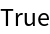The span of the second list of vectors is not contained in the span of the first list:

 In:=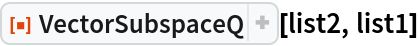Out=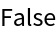Check:

The span of list1 is contained in the span of list2 since every vector in list1 is a linear combination of vectors in list2:

 In:=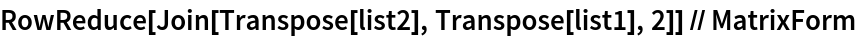Out=The first and third vectors in list2 are not linear combinations of the vectors in list1:

 In:=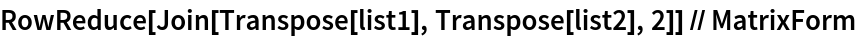Out=### Scope (5)

#### Matrices (3)

The subspace spanned by the first list of vectors (matrices) is contained in the subspace spanned by the second list:

 In:=In:=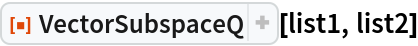Out=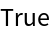However, the two subspaces are not equal:

 In:=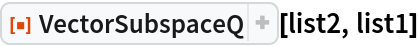Out=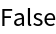Confirm by checking with the resource function SameSpanQ:

 In:=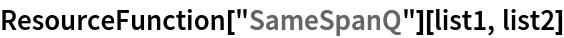Out=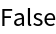#### Function Spaces (2)

The span of the first list of trigonometric functions is contained in the span of the second list, as can be verified using trigonometric identities:

 In:=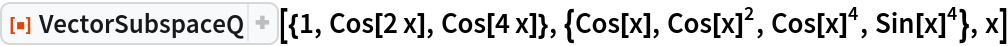Out=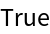But the two subspaces are not equal:

 In:=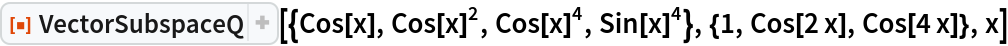Out=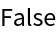The first function cos(x) is not a linear combination of 1,cos(2x),cos(4x):

 In:=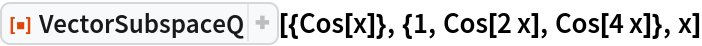Out=But each of the other functions cos2(x),cos4(x),sin4(x) in the first list is a linear combination of the three functions 1,cos(2x),cos(4x):

 In:=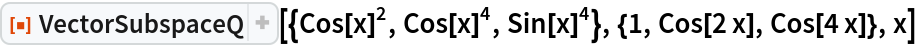Out=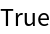The even Chebyshev polynomials of the first kind of degree at most 10 form a subspace of the space of all polynomials of degree at most 10:

 In:=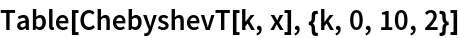Out=In:=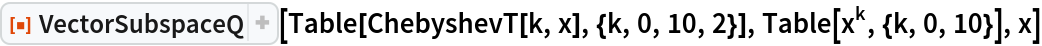Out=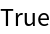But the two subspaces are not equal:

 In:=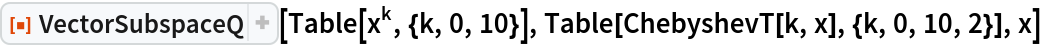Out=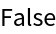Check with the resource function SameSpanQ:

 In:=Out=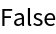## Publisher

Dennis M Schneider

## Version History

• 1.0.0 – 11 October 2019